Scan QR code or get instant email to install app

Question:

# 1212Spherical capacitance, C, is defined as the ratio of the product of 4, π, vacuum permittivity,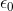, and Coulomb's constant, k to the difference between the ratio of 1 to the radial length a, and the ratio of 1 to the radial length b. Which of the following correctly solves spherical capacitance for radial length b?

A 1212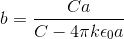explanation

1212Begin by translating the given definition into an algebraic expression.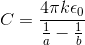Manipulate the equation to solve for b: Multiply both sides by the denominator: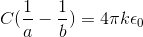, divide both sides by C: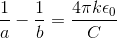, solve for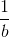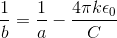, combine the right hand side using the common denominator: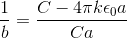, and evaluate the reciprocal of each side to find b: